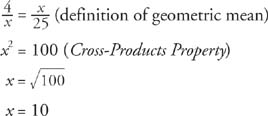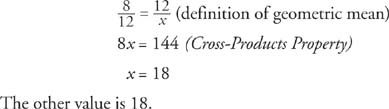## Geometric Mean

When a positive value is repeated in either the means or extremes position of a proportion, that value is referred to as a geometric mean (or mean proportional) between the other two values.

Example 1: Find the geometric mean between 4 and 25.

Let x = the geometric mean.The geometric mean between 4 and 25 is 10.

Example 2: 12 is the geometric mean between 8 and what other value?

Let x = the other value.Back to Top
A18ACD436D5A3997E3DA2573E3FD792A